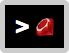# Ru## Overview

Ru brings Ruby's expressiveness, cleanliness, and readability to the command line.

It lets you avoid looking up pesky options in man pages and Googling how to write a transformation in bash that would take you approximately 1s to write in Ruby.

For example, to center a file's lines, use String#center:

``````ru 'map(:center, 80)' myfile
``````

``````awk 'printf "%" int(40+length(\$0)/2) "s\n", \$0' myfile
``````

For another example, let's compare summing the lines of a list of integers using Ru vs. a traditional approach:

``````ru 'map(:to_i).sum' myfile
``````
``````awk '{s+=\$1} END {print s}' myfile
``````

Any method from Ruby Core and Active Support can be used. Ru also provides new methods (and modifies #map) to make transformations easier. Here are some variations on the above example:

``````ru 'map(:to_i, 10).sum' myfile
ru 'map(:to_i).reduce(&:+)' myfile
ru 'each_line.to_i.to_a.sum' myfile
ru 'grep(/^\d+\$/).map(:to_i).sum' myfile
ru 'map { |n| n.to_i }.reduce(&:+)' myfile
ru 'reduce(0) { |sum, n| sum + n.to_i }' myfile
ru 'each_line.match(/(\d+)/).to_i.to_a.sum' myfile
ru 'map { |n| n.to_i }.reduce(0) { |sum, n| sum + n }' myfile
``````

See Examples and Methods for more.

## Installation

``````gem install ru
``````

You can now use Ruby in your shell!

For example, to sum a list of integers:

``````\$ printf "2\n3" | ru 'map(:to_i).sum'
5
``````

## Usage

See Examples below, too!

``````\$ printf "2\n3" | ru 'map(:to_i).sum'
5
\$ cat myfile | ru 'map(:to_i).sum'
5
``````

Or from file(s):

``````\$ ru 'map(:to_i).sum' myfile
5
\$ ru 'map(:to_i).sum' myfile myfile
10
``````

You can also run Ruby code without any input by prepending a `!`:

``````\$ ru '! 2 + 3'
5
``````

The code argument is run as if it has `\$stdin.each_line.map(&:chomp).` prepended to it. The result is converted to a string and printed. So, if you run `ru 'map(&:to_i).sum'`, you can think of it as running `puts \$stdin.each_line.map(&:chomp).map(&:to_i).sum`.

In addition to the methods provided by Ruby Core and Active Support, Ru provides other methods for performing transformations, like `each_line`, `files`, and `grep`, and it improves `map`. See Methods for more.

## Examples

Let's compare the readability and conciseness of Ru relative to existing tools:

#### Center lines

##### ru
``````ru 'map(:center, 80)' myfile
``````
##### awk
``````awk 'printf "%" int(40+length(\$0)/2) "s\n", \$0' myfile
``````

Script

#### Sum a list of integers

##### ru
``````ru 'map(:to_i).sum' myfile
``````
##### awk
``````awk '{s+=\$1} END {print s}' myfile
``````
##### paste
``````paste -s -d+ myfile | bc
``````
##### ru
``````ru '' myfile
``````
##### sed
``````sed '5q;d' myfile
``````
##### ru
``````ru '[1..-2]' myfile
``````
##### sed
``````sed '1d;\$d' myfile
``````

#### Sort an Apache access log by response time (decreasing, with time prepended)

##### ru
``````ru 'map { |line| [line[/(\d+)( ".+"){2}\$/, 1].to_i, line] }.sort.reverse.map(:join, " ")' access.log
``````
##### awk
``````awk --re-interval '{ match(\$0, /(([^[:space:]]+|\[[^\]]+\]|"[^"]+")[[:space:]]+){7}/, m); print m, \$0 }' access.log | sort -nk 1
``````

Source

## Methods

In addition to the methods provided by Ruby Core and Active Support, Ru provides other methods for performing transformations.

#### each_line

Provides a shorthand for calling methods on each iteration of the input. Best explained by example:

``````ru 'each_line.strip.center(80)' myfile
``````

If you'd like to transform it back into a list, call `to_a`:

``````ru 'each_line.strip.to_a.map(:center, 80)' myfile
``````

#### files

Converts the lines to `Ru::File` objects (see Ru::File below).

``````\$ printf "foo.txt" | ru 'files.map(:updated_at).map(:strftime, ""%Y-%m-%d")'
2014-11-08
``````

#### format(format='l')

Formats a list of `Ru::File`s. You'll typically call this after calling `files` to transform them into strings:

``````\$ ru 'files.format'
644 tom staff   3   2014-10-26  09:06   bar.txt
644 tom staff   11  2014-11-04  08:29   foo.txt
``````

The default format, `'l'`, is shown above. It prints `[omode, owner, group, size, date, name]`.

#### grep

Selects lines which match the given regex.

``````\$ printf "john\npaul\ngeorge" | ru 'grep(/o[h|r]/)'
john
george
``````

#### map

This is the same as Array#map, but it adds a new syntax that allows you to easily pass arguments to a method. For example:

``````\$ printf "john\npaul" | ru 'map(:[], 0)'
j
p
\$ printf "john\npaul" | ru 'map(:center, 8, ".")'
..john..
..paul..
``````

Note that the examples above can also be performed with `each_line`:

``````\$ printf "john\npaul" | ru 'each_line'
\$ printf "john\npaul" | ru 'each_line.center(8, ".")'
``````

## Ru::File

The `files` method returns an enumerable of `Ru::File`s, which are similar to Ruby Core's `File`. Each one has the following methods:

• `basename`
• `created_at` (alias for ctime)
• `ctime`
• `extname`
• `format` (see the `format` method above)
• `ftype`
• `gid`
• `group`
• `mode`
• `mtime`
• `name` (alias for basename)
• `omode`
• `owner`
• `size`
• `to_s` (alias for name)
• `uid`
• `updated_at` (alias for mtime)
• `world_readable?`

## Saved Commands

Ru lets you save commands by name, so that you can easily use them later.

#### save

Save a command for future use:

``````\$ ru save sum 'map(:to_i).sum'
Saved command: sum is 'map(:to_i).sum'
``````

#### run

Run a saved command:

``````\$ printf "2\n3" | ru run sum
5
\$ ru run sum myfile
5
``````

#### list

List all of your saved commands:

``````\$ ru list
Saved commands:
sum map(:to_i).sum
``````

## Options

#### -h, --help

Print a help page.

#### -v, --version

Print the installed version of Ru.

## Testing

Ru is tested against Active Support 3 and 4. If you'd like to submit a PR, please be sure to use Appraisal to test your changes in both contexts:

``````appraisal rspec
``````